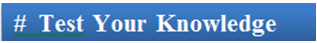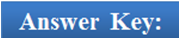×#### Thank you for registering.

One of our academic counsellors will contact you within 1 working day.

Click to Chat

1800-1023-196

+91-120-4616500

CART 0

• 0

MY CART (5)

Use Coupon: CART20 and get 20% off on all online Study Material

ITEM
DETAILS
MRP
DISCOUNT
FINAL PRICE
Total Price: Rs.

There are no items in this cart.
Continue Shopping```Differentiation of a Function Given in Parametric Form

Table of Content

Differentiation of a Function Given in Parametric Form

Differentiation of one function with respect to another function

Higher Order Derivatives

Related Resources

Differentiation of a Function Given in Parametric FormThere may at times arise situations wherein instead of expressing a function say y(x) in terms of an independent variable x only, it is convenient or advisable to express both the functions in terms of a third variable (say) t. This ‘t’ is termed as the parameter. The relationship between the two variables is so complicated that it becomes indispensable to introduce a third variable of such a type that both the variables can be represented in terms of this third variable.

Suppose there is a curve which is represented by the equations x = sin t and y = cos t. Then if x = f(t) and y = g(t) then we have

dy/dx = dy/dt . dt/dx

But  dt/dx = 1/(dx/dt)

So, dy/dx = (dy/dt)/(dx/dt)

= g’(t)/f’(t)If x = x(t) and y = y(t), then dy/dx = (dy/dt)/(dx/dt) , provided dx/dt ≠ 0.

Illustration 1:

x = a(θ + sin θ), y = a(1 – cos θ) where θ is a parameter, then find dy/dx.

Solution:

dy/dx = (dy/dθ)/(dx/dθ)

= (a sinθ)/(a (1 + cos θ))

= (2sin θ/2 cos θ/2)/(2 cos2 θ/2)

= tan θ/2.

_____________________________________________________________________

Illustration 2:

For the curve represented parametrically by the given equations, indicate the relation between the parameter t and the angle α between the tangent to the given curve and the x-axis.

x = cos t + t sin t – t2/2 cos t

and y = sin t – t cos t – t2/2 sin t

Solution:

x = cos t + t sin t – t2/2 cos t

dx/dt = – sin t + t cos t + sin t – (t2/2 sin t + t cos t)

= t2 sint /2

y = sin t – t cos t – t2/2 sin t

dy/dt = cos t – (cos t – t sin t) – ½ (2t sin t + t2 cos t) = – (t2 cos t)/2

Hence, dy/dx = (dy/dt)/(dx/dt) = – cos t = tan α

Hence, this gives, tan (π/2– t) = tan (-α)

This gives π/2 – t = – α

which gives t = π/2 + α.

Differentiation of one function with respect to another function

If y = f(x) and z = g(x) then the derivative of f(x) with respect to g(x) is given by

dy/dz = dy/dx . dx/dz

= f’(x)/g’(x)

Hence, the differential coefficient of f(x) with respect to g(x) is given by

= (derivative of f(x) with respect to x)/(derivative of g(x) with respect to x)

= f’(x)/g’(x)

Illustration:

Find the derivative of (ln x)tan x with respect to xx.

Solution:

Let u = (ln x)tan x and let v = xx

We consider both the functions one by one and try solving them by taking logarithm of both the sides

Now, u = (ln x)tan x

Taking logarithm of both the sides we have,

ln u = tan x  ln (ln x)

Differentiation gives

1/u (du/dx) = (sec2x) ln (ln x) + tan x (1/ln x . 1/x)

This gives du/dx = [u {(x ln x) ln(ln x) sec2x + tan x}]/(x ln x)

Now, v = xx

Hence, ln v = x ln x

On differentiation, this gives

1/v (dv/dx) = (ln x + 1)

So, du/dx = (ln x)tan x/ xx . [{ x ln x ln (ln x) sec2x + tan x}/(x ln x)(ln x + 1)].

Note:Higher Order Derivatives

As the first derivative or the first order derivative of a function y = f(x) can be represented by the symbols dyd/x, Dy, f’(x) or simply y’, similarly, in case of higher order derivatives we have,

d2y/dx2 = d/dx (dy/dx)

d3y/dx3 = d/dx ((d2 y)/(dx2))

(dn y)/dxn = d/dx ((d(n-1) y)/dx(n-1));

Here, (dny)/dxn is called the nth order derivative of y with respect to x.A homogeneous equation of degree n represents n straight lines passing through the origin and hence dy/dx = y/x and d2y/dx2 = 0.

Eg: If we have an equation x3 + 3x2y – 6xy2 + 2y3 = 0, then the value of d2y/dx2 at the point (1,1) = 0.

Illustration:

If y = (sin-1x)2 + k sin-1x, show that (1-x2) (d2y)/dx2 – x dy/dx = 2

Solution:

Here y = (sin-1x)2 + k sin-1x.

Differentiating both sides with respect to x, we have

dy/dx = 2(sin(-1)x)/√(1-x2) + k/√(1 – x2) ⇒ (1 – x2) (dy/dx)2

=4y + k2

Differentiating this with respect to x, we get

(1 - x2) 2 dy/dx.(d2y)/(dx2) -2x (dy/dx)2 = 4(dy/dx)

⇒ (1-x2) (d2y)/dx2 – x dy/dx = 2.

_______________________________________________________________________

Illustration:

If y = e(sin2 x), find (d2 x)/dy2 , in terms of x.

Solution:

Here y = e(sin2 x). Differentiating with respect to x, we get

dy/dx = sin 2x.e(sin2 x) ⇒ dx/dy = cosec 2x.e(-sin2 x)

Differentiating with respect to y, we get

(d2 x)/dy2 = d/dy (cosec 2x.e(-sin2 x)) = d/dx (cosec 2x.e(- sin2 x)) dx/dy

= (-2 cosec 2xcot 2x e(- sin2 x) – e(-sin2x))

= (-2 cosec2 2x cot 2x + cosec 2x)e(-sin2 x)

Differential coefficient of a function y = uv where u and v are both function of x or u is a constant and v is a function of x:

Coefficient of a function of the form uv cannot be found directly by using standard formula and hence in such cases both sides are differentiated after taking logarithm. This process is called logarithmic differentiation.

Let y = uv

Taking logarithm on both side

ln y = v ln u

Differentiating, w.r.t. x, we get

1/y dy/dx = v/u du/dx + log u dv/dx

⇒ dy/dx = uv [v/u du/dx + log u dv/dx]

Illustration:

If xy = yx, find dy/dx.

Solution:

The given function is xy = yx.

taking logarithm, we get y loge x =x loge y

Differentiating w.r.t x, we get

loge x dy/dx+y.1/x = 1.loge y+x .1/y dy/dx

loge x-x/y)  dx/dy-loge y- y/x

or (y log(e x-x))/y dy/dx = (x log(e y-y))/(y loge x-x)1. If y = ax, a > 0 then dy/dx = ax loge a

2. While differentiating functions of this form it is always advisable to take natural log. Also if you have log function with base other than 'e', then convert it to the base 'e' before differentiating.

For more on differentiation of implicit functions,refer the following video

Illustration:

If y = log2 log3 log4 x, find dy/dx.

Solution:

The given function is y = log2 log3 log4 x

= (loge log3 log4 x) (log2 e)Q1. For the curve represented by x = sin t and y = cos t, dy/dx =

(a) (dy/dt)/(dx/dt)

(b) dy/dt

(c) (dy/dt)/(dx/dt), provided dx/dt ≠ 0.

(d) dx/dt

Q2. Differentiating the function uv, we get

(a) dy/dx = uv [v du/dx + log u dv/dx]

(b) dy/dx = uv [v/u du/dx + u dv/dx]

(c) dy/dx = uv [v/u du/dx + log u dv/dx]

(d) none of the above

Q3. The deirvative of even differentiable function is

(a) even function

(b) odd function

(c) may be even or odd

(d) can’t say

Q4. The differential coefficient of f(x) with respect to g(x) is given by

(a) f’(x)/g’(x)

(b) f’(x)/g(x)

(c) f’(x)/g’’(x)

(d) f(x)/g’(x)

Q5.If x = at2 and y = 2at, then dy/dx =

(a) t

(b) 1

(c) 0

(d) 1/tQ1.
Q2.
Q3.
Q4.
Q5.

(a)
(c)
(b)
(a)
(d)

Related Resources

You may also like to refer Differentiation of Composite Functions.

For getting an idea of the type of questions asked, refer the previous year papers.

To read more, Buy study materials of Methods of Differentiation comprising study notes, revision notes, video lectures, previous year solved questions etc. Also browse for more study materials on Mathematics here.
```### Course Features

• 731 Video Lectures
• Revision Notes
• Previous Year Papers
• Mind Map
• Study Planner
• NCERT Solutions
• Discussion Forum
• Test paper with Video Solution